# Detecting Voltage of System Reset IC

#### Tony9168

Joined Mar 6, 2016
32
Hi Guys,

System reset IC is used as overvoltage protection circuit in my application. The pdf datasheet of the system reset IC (JRC - NJM2102) is attached. Please check.

1. How to adjust the different set value for detecting voltage of the system reset IC? The example application in the datasheet show the table with different set voltage, however, i couldn't understand how to set it. Please advise. (Page 9 of the attached datasheet)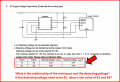2. Face some difficulty in the block diagram. (Page 1 of attached datasheet)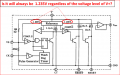#### Attachments

• 218.5 KB Views: 3

#### Alec_t

Joined Sep 17, 2013
10,690
What the datasheet is telling you (seemingly) is that R1 and R2 can be used to set the voltage and providing they are less than 1/10 of the internal resistor values you can, to a first approximation, ignore those internal resistors. In reality R1 will be in parallel with 100kΩ and R2 will be in parallel with 40kΩ.
So, you choose R1 and R2 such that Vset*R2/(R1+R2) = 1.235V.
The reference voltage (1.235V) is independent of the IC supply voltage (assumed within the recommended range).
Note that there is a slight hysteresis around Vset.

•Tony9168

#### Tony9168

Joined Mar 6, 2016
32
What the datasheet is telling you (seemingly) is that R1 and R2 can be used to set the voltage and providing they are less than 1/10 of the internal resistor values you can, to a first approximation, ignore those internal resistors. In reality R1 will be in parallel with 100kΩ and R2 will be in parallel with 40kΩ. [Thanks for your explanation. Understand]

So, you choose R1 and R2 such that Vset*R2/(R1+R2) = 1.235V.
The reference voltage (1.235V) is independent of the IC supply voltage (assumed within the recommended range).
Note that there is a slight hysteresis around Vset.
[Still got doubt]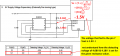#### Jony130

Joined Feb 17, 2009
5,123
Simply for Vs = 5V you do not get the reset because pin 7 voltage is higher than 1.235V. So where do you see a problem?

#### Jony130

Joined Feb 17, 2009
5,123
For R1 = 9.1kΩ and R2 = 3.9kΩ the supply voltage must drop bellow 1.235V * (1 + R1/R2) ≈ 4.11V minus the hysteresis (0.1V) to get the reset signal active.

Last edited:
•Tony9168

#### bertus

Joined Apr 5, 2008
20,226
Hello,

The external voltage divider is parallel with the internal voltage divider of 100K / 40K.
That will shift the value a bit.

Bertus

#### Tony9168

Joined Mar 6, 2016
32
For R1 = 9.1kΩ and R2 = 3.9kΩ the supply voltage must drop bellow 1.235V * (1 + R1/R2) ≈ 4.11V minus the hysteresis (0.1V) to get the reset signal active.
Hi Jony, thanks for the example shown. My understanding is the set voltage (Vset) is nothing related with the supply voltage. And please check whether my calculation of the set voltage for the example below is correct?

How is this formulae <<1.235V * (1 + R1/R2) ≈ 4.11V>> derived? Is there any material to enable more understanding? Please intro. Thanks.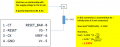#### Tony9168

Joined Mar 6, 2016
32
Hello,

The external voltage divider is parallel with the internal voltage divider of 100K / 40K.
That will shift the value a bit.

Bertus
Thanks for the reminder.

Off topic: I like your quote!

When you do ask questions, you may look stupid.
When you do NOT ask questions, you will STAY stupid.

Cheers.#### Jony130

Joined Feb 17, 2009
5,123
My understanding is the set voltage (Vset) is nothing related with the supply voltage
Unfortunately, I must disagree with you. The main purpose of this IC is to detect the supply voltage drop or some other voltage line/rail drop below Vset value.

How is this formulae <<1.235V * (1 + R1/R2) ≈ 4.11V>> derived?
It comes directly from the voltage divider equation: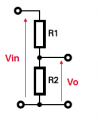We have

Vo = I*R2 and I = Vin/(R1 + R2) hence :

Vo = I*R2 = Vin/(R1 + R2) * R2 = Vin * R2/(R1 + R2)

Vo = Vin * R2/(R1 + R2)

Vo/Vin = R2/(R1 + R2)

Vin/Vo = (R1 + R2)/R2

Vin/Vo = R1/R2+ R2/R2

Vin/Vo = 1 + R1/R2

Vin = Vo* 1 + R1/R2

And in this IC we have Vo = 1.235V

And please check whether my calculation of the set voltage for the example below is correct?
Without R2 we have this situation

Vset = 1.235V * 1 + (1kΩ||100kΩ)/40kΩ ≈ 1.235V * (1 + 1kΩ/40kΩ) ≈ 1.26V

#### Tony9168

Joined Mar 6, 2016
32
Unfortunately, I must disagree with you. The main purpose of this IC is to detect the supply voltage drop or some other voltage line/rail drop below Vset value.

It comes directly from the voltage divider equation:

View attachment 170387

We have

Vo = I*R2 and I = Vin/(R1 + R2) hence :

Vo = I*R2 = Vin/(R1 + R2) * R2 = Vin * R2/(R1 + R2)

Vo = Vin * R2/(R1 + R2)

Vo/Vin = R2/(R1 + R2)

Vin/Vo = (R1 + R2)/R2

Vin/Vo = R1/R2+ R2/R2

Vin/Vo = 1 + R1/R2

Vin = Vo* 1 + R1/R2

And in this IC we have Vo = 1.235V

Without R2 we have this situation

Vset = 1.235V * 1 + (1kΩ||100kΩ)/40kΩ ≈ 1.235V * (1 + 1kΩ/40kΩ) ≈ 1.26V

Thanks. Understand.